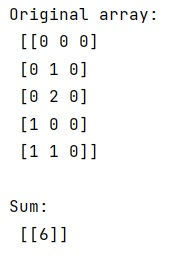# What does the 'keepdims' parameter do with numpy.sum() function?

Learn what does the 'keepdims' parameter do with numpy.sum() function in Python?
Submitted by Pranit Sharma, on February 11, 2023

NumPy is an abbreviated form of Numerical Python. It is used for different types of scientific operations in python. Numpy is a vast library in python which is used for almost every kind of scientific or mathematical operation. It is itself an array which is a collection of various methods and functions for processing the arrays.

## Usages of 'keepdims' parameter with numpy.sum() function

The numpy.sum() is used to sum of array elements over a given axis. It takes a lot of parameters like dtype, out, initial, and keepdims.

keepdims is a boolean type parameter, if this is set to True, the axes which are reduced are left in the result as dimensions with size one. With this option, the result will broadcast correctly against the input array.

If the default value is passed, then keepdims will not be passed through to the sum method of sub-classes of ndarray, however, any non-default value will be. If the sub-class' method does not implement keepdims any exceptions will be raised.

Let us understand with the help of an example,

## Python code to demonstrate the use of 'keepdims' parameter with numpy.sum() function

```# Import numpy
import numpy as np

# Creating a numpy array
arr = np.array([[0, 0, 0],
[0, 1, 0],
[0, 2, 0],
[1, 0, 0],
[1, 1, 0]])

# Display original array
print("Original array:\n",arr,"\n")

# Calculating sum
res = np.sum(arr, keepdims=True)

# Display result
print("Sum:\n",res)
```

Output:As we can see, by passing keepdims=True, the final result has the retained dimensions.